Homework Explained - Math Practice 101Dear guest, you are not a registered member. As a guest, you only have read-only access to our books, tests and other practice materials.

As a registered member you can:

Registration is free and doesn't require any type of payment information. Click here to Register.
Go to page:
Chapter 10:Volume and Surface Area;Lesson 1:Volume of Rectangular Prisms

### Independent Practice

Find the volume of each prism.

• Question 1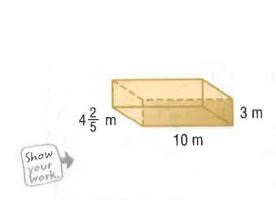•  $$V=$$ $$m^3$$
• Question 2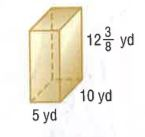• $$V=$$
• $$yd^3$$
• Question 3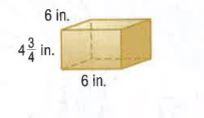•  $$V=$$ $$in.^3$$
• Question 4

A fishing tackle box is 13 inches long, 6 inches wide, and $$2\frac{1}{2}$$ inches high.
What is the volume of the tackle box?

•  V = in.³
• Question 5

Find the length of a rectangular prism having a volume of 2,830.5 cubic meters, width of 18.5 meters, and height of 9 meters.

•  l = meters

Find the missing dimension of each prism.

• Question 6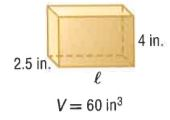•  $$l=$$ in.
• Question 7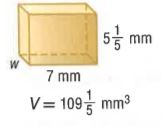•  w = mm
• Question 8

In Japan, farmers have created watermelons in the shape of rectangular prisms. Find the volume of a prism -shaped watermelon in cubic inches if its length is 10 inches, its width is $$\frac{2}{3}$$foot, and its height is 9 inches.

•  V = cubic inches
• Question 9

The glass container shown is filled to a height of 2.25 inches.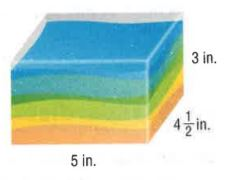a. How much sand is currently in the container?

•  cubic in.
• Question 9

b. How much more sand could the container hold before it overflows?

•  cubic in.
• Question 9

c. What percent of the container is filled with sand?

•  $$\%$$

Yes, email page to my online tutor. (if you didn't add a tutor yet, you can add one here)